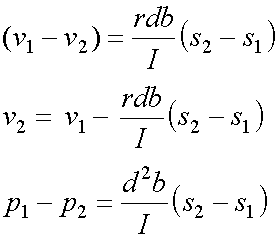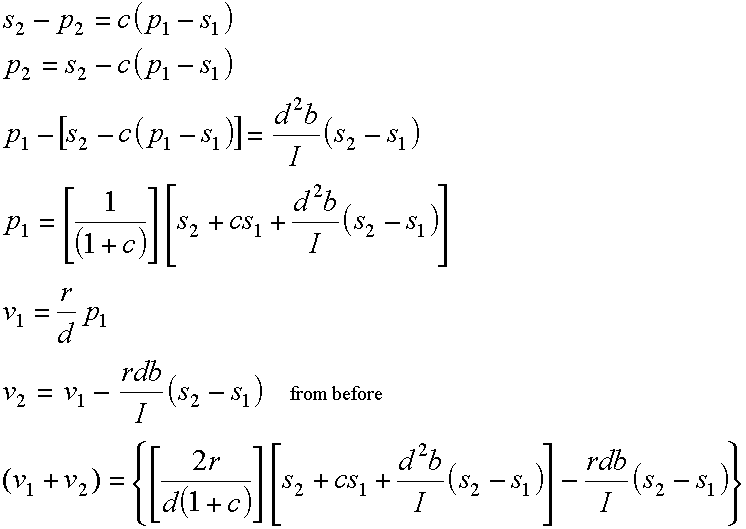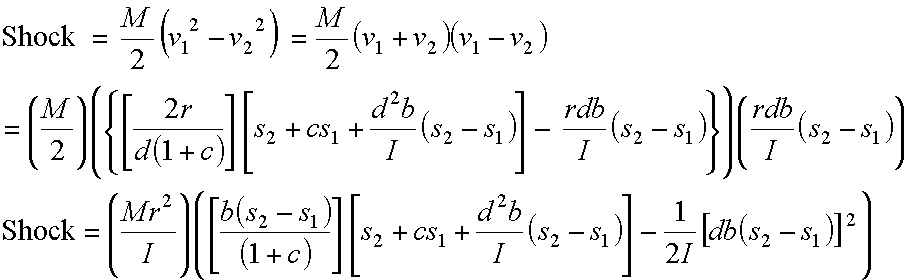## Derivation of the Shock Formula

Shock is the difference between the initial and final kinetic energy of the racquet. See the accompanying remarks on this derivation. In general, the total kinetic energy of a rigid body in plane motion (e.g. a swinging racquet) is the sum of: (1) the kinetic energy associated with the linear velocity of the mass center [½ Mv²] and (2) the kinetic energy associated with its rotation about the axis of rotation [½ Iw²]. The first component is due to body rotation, arm swing, etc., and is assumed to be the same for all racquets used by the same player under the two Benchmark Conditions. Over the course of the impact, the before vs. after difference in linear velocity of the mass center associated with this first component alone will be negligibly small anyway. Any difference will come as the racquet’s rotation slows down or speeds up during the impact. So the first component is disregarded, and the second component is what is concerned in the following derivation. However, the second component (rotational kinetic energy) is converted into kinetic energy associated with the linear velocity of the mass center so as to be expressible in joules. A complete legend of variables used follows after the derivation.

The first step follows from the Torque derivation: